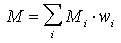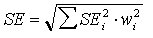## 2.4. Stratified sampling

Stratified sampling is used if sampled area (or volume) is heterogeneous (Pielou, p.107). If patch size is much larger than inter-sample distance, then kriging can be used instead of stratified sampling.

In stratified sampling program, the area (volume) is subdivided into 2 or more portions which are sampled separately. Example: pine sawflies prefer to spin their cocoons close to the tree; thus, the area adjacent to trees (within 1 m radius) can be sampled separately from the rest of the area.

1. Density of samples in each stratum should be proportional to the variance of organism counts in the stratum -- in this case the maximum precision is reached. Taylor's power law can be used to predict variance from the mean. Variance usually increases with mean, and thus, the stratum with higher organism density should be sampled more intensively.

2. The mean population density, M, is estimated as a weighted mean of mean densities, Mi, in each stratum, i, with weights, wi equal to the area covered by stratum i:3. The standard error, SE, of the mean is equal towhere SEi is the standard error for the mean in stratum i.

Alexei Sharov 1/12/96